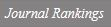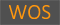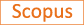Existence of nontrivial weak solutions for a quasilinear Choquard equation

Title
Existence of nontrivial weak solutions for a quasilinear Choquard equation
Authors
Lee J.Kim J.-M.Bae J.-H.Park K.
Ewha Authors
Issue Date
2018
Journal Title
Journal of Inequalities and Applications
ISSN
1025-5834Citation
Journal of Inequalities and Applications vol. 2018
Keywords
Choquard equationVariational methodWeak solutions
Publisher
Springer International Publishing
Indexed
SCOPUSDocument Type
Article
Abstract
We are concerned with the following quasilinear Choquard equation: −Δpu+V(x)

u

p−2u=λ(Iα∗F(u))f(u)in RN,F(t)=∫0tf(s)ds,(Formula presented.) where 1 &lt; p&lt; ∞ , Δ pu= ∇ ⋅ (

∇ u

p − 2∇ u) is the p-Laplacian operator, the potential function V: RN→ (0 , ∞) is continuous and F∈ C1(R, R). Here, Iα: RN→ R is the Riesz potential of order α∈ (0 , p). We study the existence of weak solutions for the problem above via the mountain pass theorem and the fountain theorem. Furthermore, we address the behavior of weak solutions to the problem near the origin under suitable assumptions for the nonlinear term f. © 2018, The Author(s).
DOI
10.1186/s13660-018-1632-z
Appears in Collections:
연구기관 > 수리과학연구소 > Journal papers
Files in This Item:
There are no files associated with this item.
Export
RIS (EndNote)
XLS (Excel)
XML